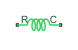# Rotational Spring

Ideal spring in mechanical rotational systems

## Library

Mechanical Rotational Elements

•## Description

The Rotational Spring block represents an ideal mechanical rotational linear spring, described with the following equations:

`$T=K·\phi$`
`$\phi ={\phi }_{init}+{\phi }_{R}-{\phi }_{C}$`
`$\omega =\frac{d\phi }{dt}$`

where

 `T` Torque transmitted through the spring `K` Spring rate φ Relative displacement angle (spring deformation) φinit Spring preliminary winding (spring offset) φR, φC Absolute angular displacements of terminals R and C, respectively ω Relative angular velocity `t` Time

The block positive direction is from port R to port C. This means that the torque is positive if it acts in the direction from R to C.

### Variables

To set the priority and initial target values for the block variables prior to simulation, use the Initial Targets section in the block dialog box or Property Inspector. For more information, see Set Priority and Initial Target for Block Variables.

Nominal values provide a way to specify the expected magnitude of a variable in a model. Using system scaling based on nominal values increases the simulation robustness. Nominal values can come from different sources, one of which is the Nominal Values section in the block dialog box or Property Inspector. For more information, see Modify Nominal Values for a Block Variable.

## Parameters

Spring rate

Spring rate. The default value is `10` N*m/rad.

## Ports

The block has the following ports:

`R`

Mechanical rotational conserving port.

`C`

Mechanical rotational conserving port.

## Version History

Introduced in R2007a